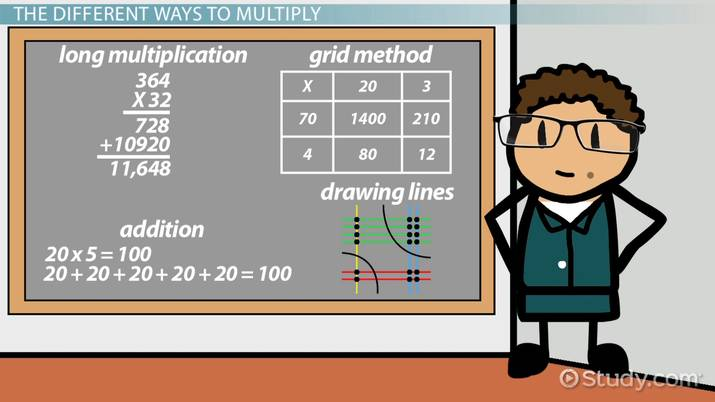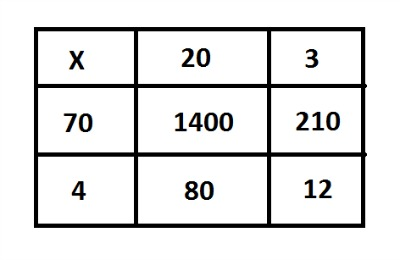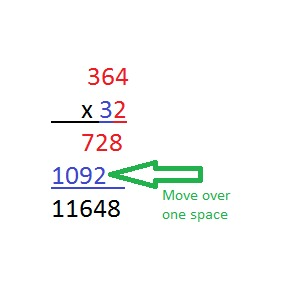# What Are Different Ways to Multiply?

Lesson Transcript
Instructor: Cori Hodges

Cori has degrees in Elementary Education and Early Childhood Education and has taught lower elementary.

Did you know there is more than one way to multiply numbers? In this lesson, we'll talk about some different ways to multiply to help you find the one that is easiest for you.

## The Different Ways to Multiply

This lesson will cover four different ways to multiply numbers: addition, memorizing the grid method, long multiplication, and drawing lines. Learning about each of them will help you decide which method works best for you.An error occurred trying to load this video.

Try refreshing the page, or contact customer support.

Coming up next: Different Ways to Divide

### You're on a roll. Keep up the good work!

Replay
Your next lesson will play in 10 seconds
• 0:00 The Different Ways to Multiply
• 0:42 The Grid Method
• 1:37 The Long Multiplication Method
• 2:22 The Drawing Lines Method
• 3:15 Lesson Summary
Save Save

Want to watch this again later?

Timeline
Autoplay
Autoplay
Speed Speed

One of the most basic ways to multiply is to add. When we multiply two numbers, we're simply adding one number to itself a certain number of times. For example, if the problem says to multiply 20 x 5, you're adding the number 20 to itself five times. So:

20 + 20 + 20 + 20 + 20 = 100

Or:

20 x 5 = 100

## The Grid MethodWhen using grid multiplication, you separate your numbers into boxes. The problem in this grid is asking you to multiply 23 x 74. In the top row, the number '23' appears as '20' and '3'. In the first column on the left, the number '74' appears as '70' and '4'.

Multiply the first number in the top row by the first number in the first column:

20 x 70 = 1,400

Then multiply the next number in the top row by 70:

3 x 70 = 210

Next, multiply the first number in the top row by the second number in the first column:

20 x 4 = 80

Finally, multiply 3 x 4 = 12.

1,400 + 210 + 80 + 12 = 1,702

## The Long Multiplication Method

Long multiplication is a way to multiply two multiple-digit numbers. For instance, let's say that a multiplication problem asks you to multiply 364 x 32. Begin by writing the problem on a piece of paper. Then multiply the top number (364) by the bottom right number (2), shown in red, and write that below the line. Now, multiply the top number (364) by the bottom left number (3), shown in blue. When you write your answer down, leave a space for a zero. Then add the two answers together to obtain your final answer:

728 + 10,920 = 11,648To unlock this lesson you must be a Study.com Member.

### Register to view this lesson

Are you a student or a teacher?